# Test: Linear Differential Equations

## 10 Questions MCQ Test Mathematics (Maths) Class 12 | Test: Linear Differential Equations

Description
This mock test of Test: Linear Differential Equations for JEE helps you for every JEE entrance exam. This contains 10 Multiple Choice Questions for JEE Test: Linear Differential Equations (mcq) to study with solutions a complete question bank. The solved questions answers in this Test: Linear Differential Equations quiz give you a good mix of easy questions and tough questions. JEE students definitely take this Test: Linear Differential Equations exercise for a better result in the exam. You can find other Test: Linear Differential Equations extra questions, long questions & short questions for JEE on EduRev as well by searching above.
QUESTION: 1

### The solution of the differential equation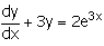is :

Solution:

dy/dx + 3y = 2e3x
p = 3,    q = 2e3x
∫p.dx = 3x
Integrating factor (I.F) = e3x
y(I.F) = ∫Q(I.F) dx
ye3x = ∫2e3x e3x dx
ye3x = ∫2e6x dx
ye3x = 2∫e6x dx
ye3x = 2/6[e6x] + c
ye3x = ⅓[e6x] + c
Dividing by e3x, we get
y = ⅓[e3x] + ce-(3x)

QUESTION: 2

### The integrating factor of differential equation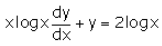is :

Solution:

xlog x dy/dx + y = 2logx
⇒ dy/dx + y/(xlogx) = 2/x...(1)
Put P = 1/x logx
⇒∫PdP = ∫1/x logx dx
= log(logx)
∴ I.F.= e∫PdP = elog(logx)
= logx

QUESTION: 3

### The solution of the differential equation x dy = (2y + 2x4 + x2) dx is:​

Solution:

xdy = (2y + 2x4 + x2)dx
→ dy/dx − (2x)y = 2x3 + x
This differential is of the form y′+P(x)y=Q(x) which is the general first order linear differential equation, where P(x) and Q(x) are continuous function defined on an interval.
The general solution for this is y∙I.F = ∫I.F × Q(x)dx
Where I.F = e∫P(x)dx is the integrating factor of the differential equation.
I.F = e∫P(x)dx
= e∫−2/xdx
= e(−2∙lnx)
= eln(x−2)
= x−2
Thus y(1/x2) = ∫1/x2(2x3 + x)dx
=∫(2x + 1/x)dx
= x2 + lnx + C
⟹ y = x4 + x2lnx + c

QUESTION: 4

The solution of the differential equation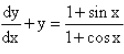Solution:
QUESTION: 5

The integrating factor of differential equation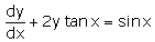is :

Solution:

dy/dx+2ytanx=sinx
This is in the form of dy/dx + py = θ
where p=2tanx,θ=sinx
∴ finding If e∫pdx = e∫2tanxdx
=e(2log secx)
=e(log sec2x)
=sec2x

QUESTION: 6

The solution of the differential equation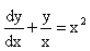is :

Solution:

dy/dx + y/x = x2
differential equation is in the form : dy/dx + Py = Q
P = 1/x      Q = x2
I.F = e∫P(x)dx
I.F = e∫1/x dx
I.F = e[log x]
I.F = x
y I.F = ∫(Q * I.F) dx + c
yx = ∫x2 * x * dx + c
yx = ∫x3 dx + c
xy = [x4]/4 + c

QUESTION: 7

The solution of the differential equation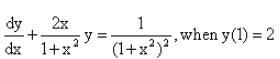is :

Solution:
QUESTION: 8

The solution of the differential equation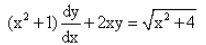is :

Solution:

The given differential equation may be written as
dy/dx + (2x/(x2+1)y = √x2+4/(x2+1)  ... (i)
This is of the form dy/dx + Py = Q,
where P=2x/(x2+1) and Q=(√x2+4)/(x2+1)
Thus, the given differential equation is linear.
IF=e(∫Pdx)
= e(∫2x(x2+1)dx)
= e(log(x2+1) = (x2+1)
So, the required solution is given by
y × IF = ∫{Q×IF}dx + C,
i.e., y(x2+1)=∫(√x2+4)/(x2+1)×(x2+1)dx
⇒y(x2+1)=∫(√x2+4)dx
=1/2x (√x2+4) +1/2 × (2)2 × log|x+(√x2+4)| + C
=1/2x (√x2+4) + 2log|x+(√x2+4) + C.
Hence, y(x2+1) = 1/2x(√x2+4) + 2log|x+√x2+4| + C is the required solution.

QUESTION: 9

The integrating factor of differential equation ​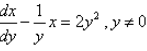is :

Solution:
QUESTION: 10

The integrating factor of differential equation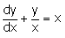is :​

Solution:

dy/dx + y/x = x
Differential eq is in the form of : dy/dx + Py = Q
P = 1/x     Q = x
I.F. = e∫Pdx
= e∫(1/x)dx
= e(log x)
⇒ x